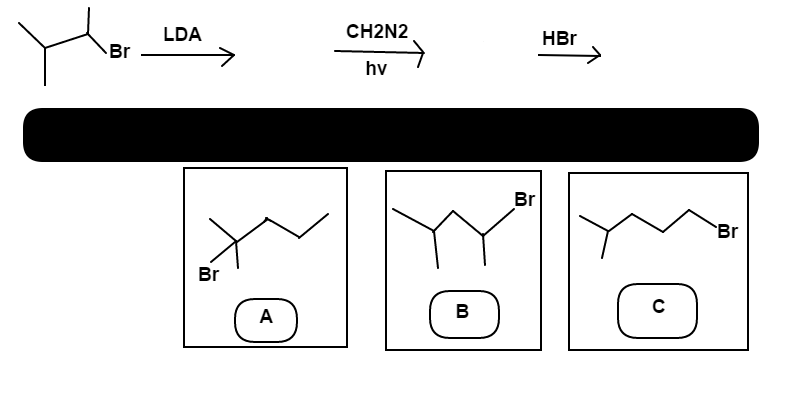# Bromo-migration

Chemistry Level 3$\text{2-bromo-3-methyl~butane}$ is reacted first with $\text{Lithium~Di-isopropylamide}$. Next, $\text{Diazomethane}$ is added while the reaction vessel was exposed to $\ce{light}$. Finally, $\ce{Hydrobromic~acid}$ is mixed into the reaction vessel.

Determine which alkyl halide; $A$, $B$, or $C$, is the major product of this reaction.

David's Organic Chemistry Set

David's Physical Chemistry Set

×